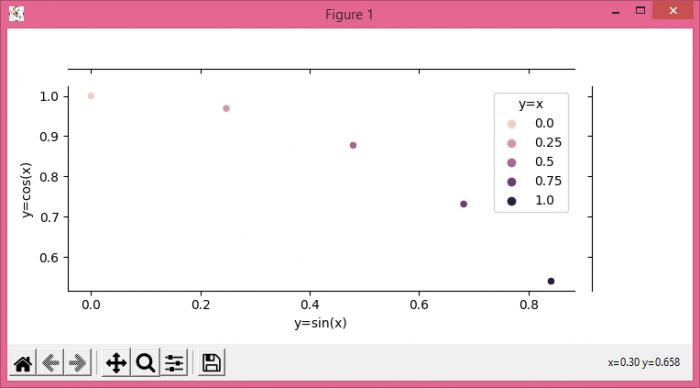# How to plot a jointplot with 'hue' parameter in Seaborn? (Matplotlib)

MatplotlibPythonData Visualization

To plot a jointplot with hue parameter in Seaborn, we can take the following steps −

## Steps

• Set the figure size and adjust the padding between and around the subplots.
• Create x data points using numpy.
• Make a dictionary with some curve data.
• Make a dataframe for tabular data.
• Make a jointplot using jointplot() method.
• To display the figure, use show() method.

## Example

from matplotlib import pyplot as plt
import pandas as pd
import seaborn as sns
import numpy as np

plt.rcParams["figure.figsize"] = [7.50, 3.50]
plt.rcParams["figure.autolayout"] = True

x = np.linspace(0, 1, 5)

d = {
'y=sin(x)': np.sin(x),
'y=cos(x)': np.cos(x),
'y=x': x,
}
df = pd.DataFrame(d)
jg = sns.jointplot(data=df, x="y=sin(x)", y="y=cos(x)",
height=3.5, hue="y=x")
plt.show()

## Output